# Electricity Lecture Series Electric Force Electric Field Applied

• Slides: 20Electricity Lecture Series Electric Force & Electric Field Applied Sciences Education Research Group (ASERG) Faculty of Applied Sciences Universiti Teknologi MARA email: [email protected] com [email protected] uitm. edu. my; http: //www 3. uitm. edu. my/staff/drjj/ Copyright DR JJ, FSG, Ui. TM 1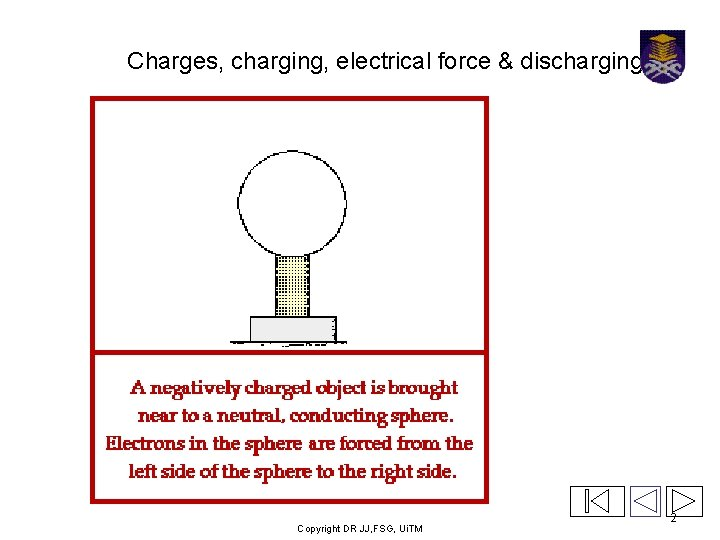Charges, charging, electrical force & discharging Copyright DR JJ, FSG, Ui. TM 2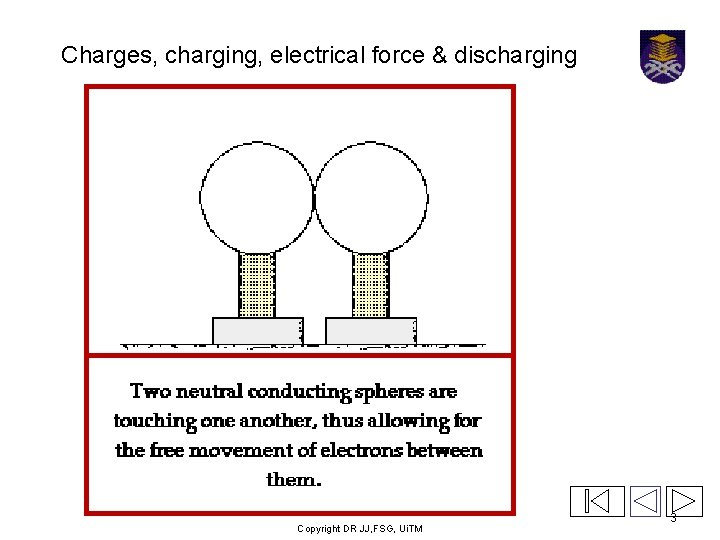Charges, charging, electrical force & discharging Copyright DR JJ, FSG, Ui. TM 3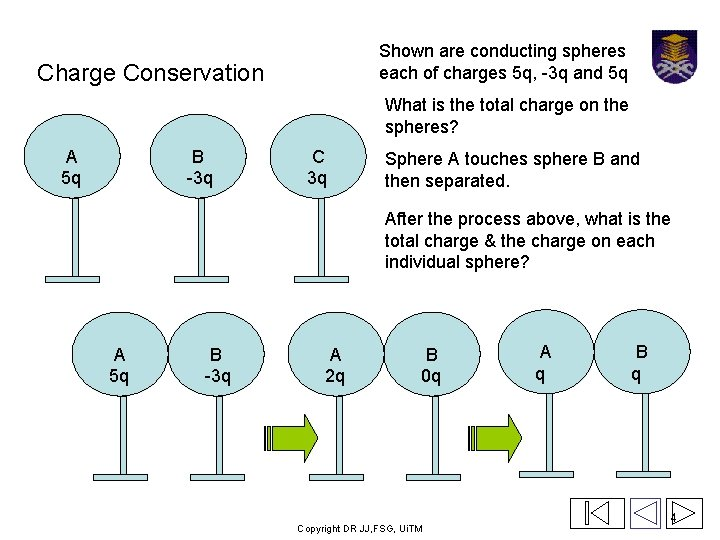Shown are conducting spheres each of charges 5 q, -3 q and 5 q Charge Conservation What is the total charge on the spheres? A 5 q B -3 q C 3 q Sphere A touches sphere B and then separated. After the process above, what is the total charge & the charge on each individual sphere? A 5 q B -3 q A 2 q B 0 q Copyright DR JJ, FSG, Ui. TM A q B q 4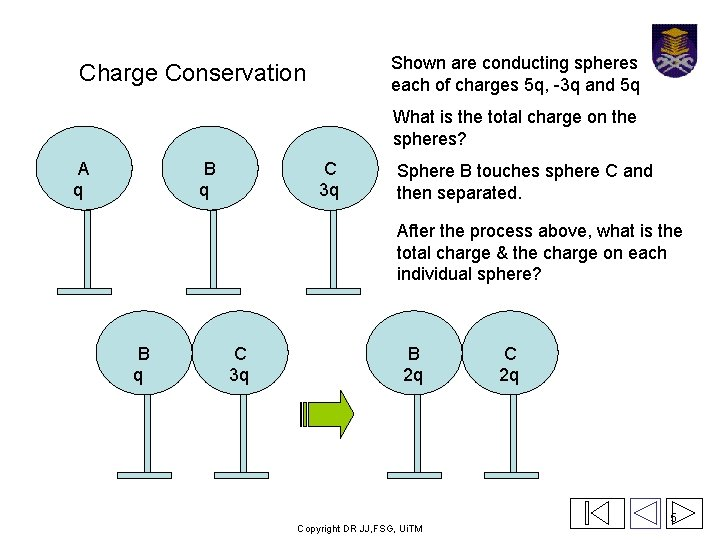Shown are conducting spheres each of charges 5 q, -3 q and 5 q Charge Conservation What is the total charge on the spheres? A q B q C 3 q Sphere B touches sphere C and then separated. After the process above, what is the total charge & the charge on each individual sphere? B q C 3 q B 2 q Copyright DR JJ, FSG, Ui. TM C 2 q 5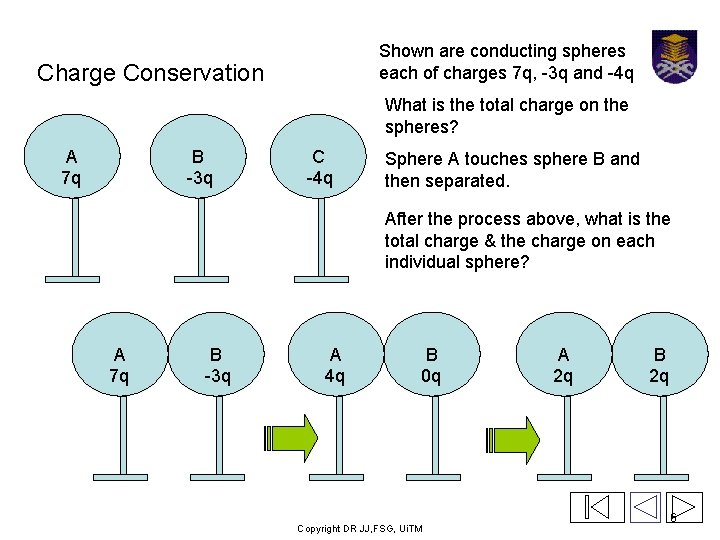Shown are conducting spheres each of charges 7 q, -3 q and -4 q Charge Conservation What is the total charge on the spheres? A 7 q B -3 q C -4 q Sphere A touches sphere B and then separated. After the process above, what is the total charge & the charge on each individual sphere? A 7 q B -3 q A 4 q B 0 q Copyright DR JJ, FSG, Ui. TM A 2 q B 2 q 6Shown are conducting spheres each of charges 2 q, 2 q and -4 q Charge Conservation What is the total charge on the spheres? A 2 q B 2 q C -4 q Sphere B touches sphere C and then separated. After the process above, what is the total charge & the charge on each individual sphere? B 2 q C -4 q B 0 q C -2 q Copyright DR JJ, FSG, Ui. TM A -q B -q 7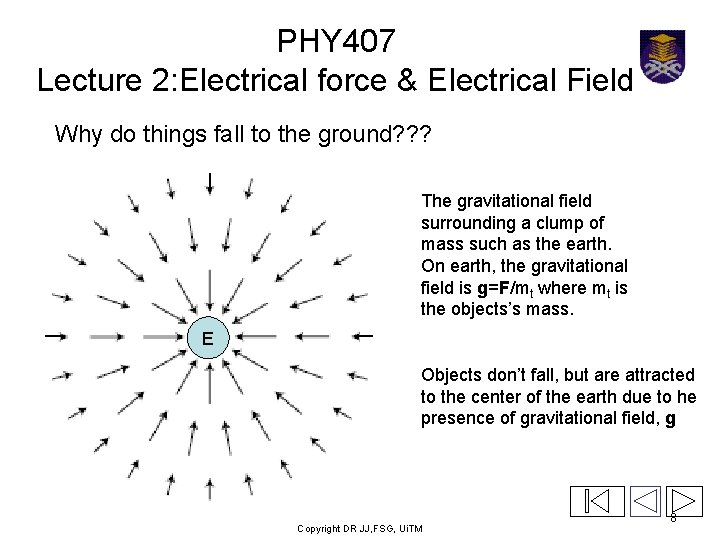PHY 407 Lecture 2: Electrical force & Electrical Field Why do things fall to the ground? ? ? The gravitational field surrounding a clump of mass such as the earth. On earth, the gravitational field is g=F/mt where mt is the objects’s mass. E Objects don’t fall, but are attracted to the center of the earth due to he presence of gravitational field, g Copyright DR JJ, FSG, Ui. TM 8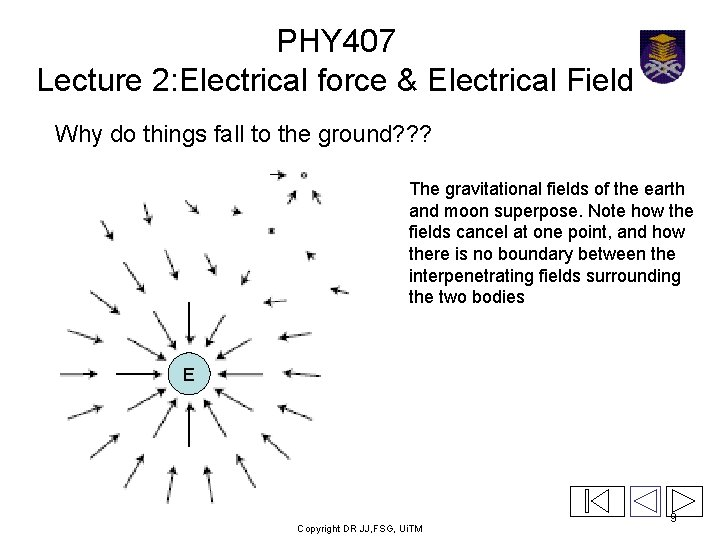PHY 407 Lecture 2: Electrical force & Electrical Field Why do things fall to the ground? ? ? The gravitational fields of the earth and moon superpose. Note how the fields cancel at one point, and how there is no boundary between the interpenetrating fields surrounding the two bodies E Copyright DR JJ, FSG, Ui. TM 9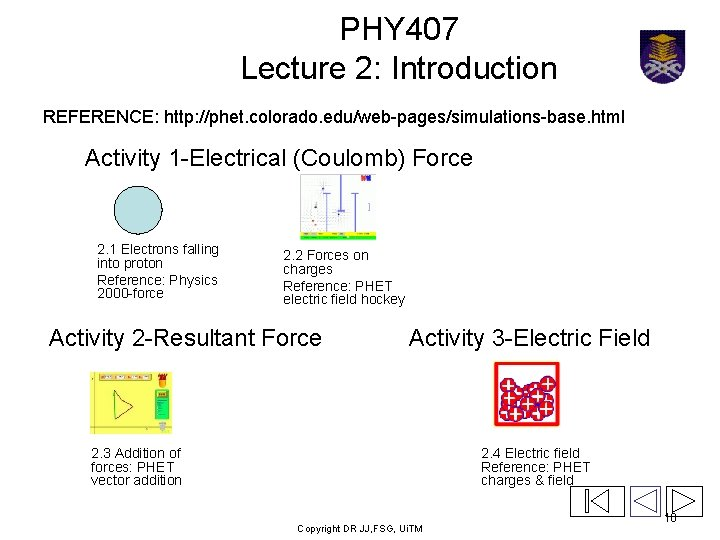PHY 407 Lecture 2: Introduction REFERENCE: http: //phet. colorado. edu/web-pages/simulations-base. html Activity 1 -Electrical (Coulomb) Force 2. 1 Electrons falling into proton Reference: Physics 2000 -force 2. 2 Forces on charges Reference: PHET electric field hockey Activity 2 -Resultant Force Activity 3 -Electric Field 2. 3 Addition of forces: PHET vector addition 2. 4 Electric field Reference: PHET charges & field Copyright DR JJ, FSG, Ui. TM 10PHY 407 Lecture 2: Introduction Activity 1 -Electrical (Coulomb) Force • Electrons move towards proton • Far electrons feel small pull, hence small initial acceleration • As the electrons accelerate and get closer, the pull gets stronger. • Near electrons feel strong pull, hence big initial acceleration. • Electrons feel the pull because they are in an electric field created by the proton + Copyright DR JJ, FSG, Ui. TM 11PHY 407 Lecture 2: Introduction Activity 1 -Electrical (Coulomb) Force • Electrons move away from negative particle • Far electrons feel small push, hence small initial acceleration • As the electrons accelerate and get further, the push gets weaker. • Near electrons feel strong push, hence big initial acceleration. • Electrons feel the push because they are in an electric field created by the negative particle _ Copyright DR JJ, FSG, Ui. TM 12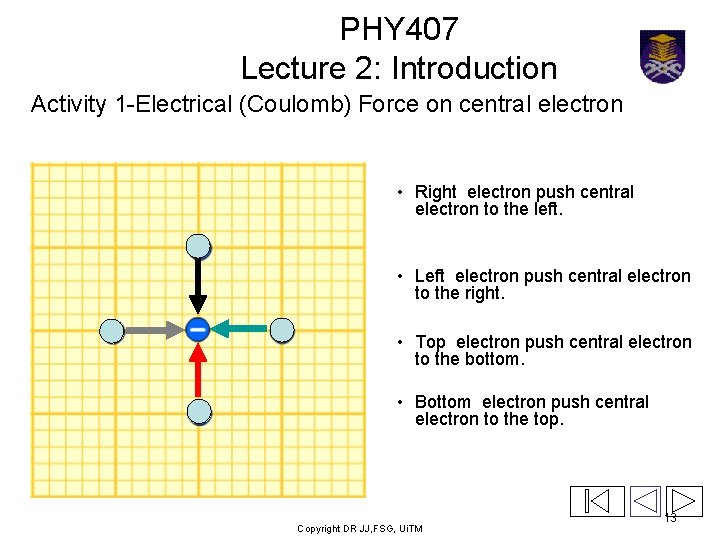PHY 407 Lecture 2: Introduction Activity 1 -Electrical (Coulomb) Force on central electron • Right electron push central electron to the left. • Left electron push central electron to the right. • Top electron push central electron to the bottom. • Bottom electron push central electron to the top. Copyright DR JJ, FSG, Ui. TM 13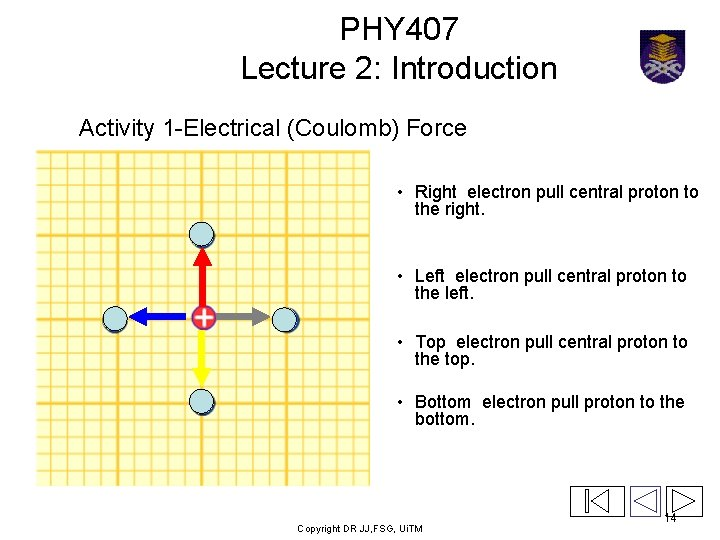PHY 407 Lecture 2: Introduction Activity 1 -Electrical (Coulomb) Force • Right electron pull central proton to the right. • Left electron pull central proton to the left. • Top electron pull central proton to the top. • Bottom electron pull proton to the bottom. Copyright DR JJ, FSG, Ui. TM 14PHY 407 Lecture 2: Introduction Activity 1 -Electrical (Coulomb) Force • Right electron pushes central electron to the left. • Left electron pushes central electron to the right with a smaller force than the electron on the right. • Top electron pushes central electron to the bottom with the same force that the left electron exerts on the central electron. • Top left corner electron pushes central electron to the bottom right corner. Copyright DR JJ, FSG, Ui. TM 15PHY 407 Lecture 2: Introduction Activity 1 -Electrical (Coulomb) Force • Right electron pulls the proton to the right. • Left electron pulls the proton to the left with a smaller force than the electron on the right. • Top electron pulls the proton up with the same force that the left electron exerts on the central electron. • Top left corner electron pulls the proton to the top left corner. Copyright DR JJ, FSG, Ui. TM 16PHY 407 Lecture 2: Introduction Activity 2 -Resultant Force PULLING of PROTON • Sum of force to the right (+ve) is equal to the sum of the force to the left (-ve). • Sum of force to the top (+ve) is equal to the sum of the force to the bottom (-ve). • Top electron push central electron to the bottom. • Bottom electron push central electron to the top. Copyright DR JJ, FSG, Ui. TM 17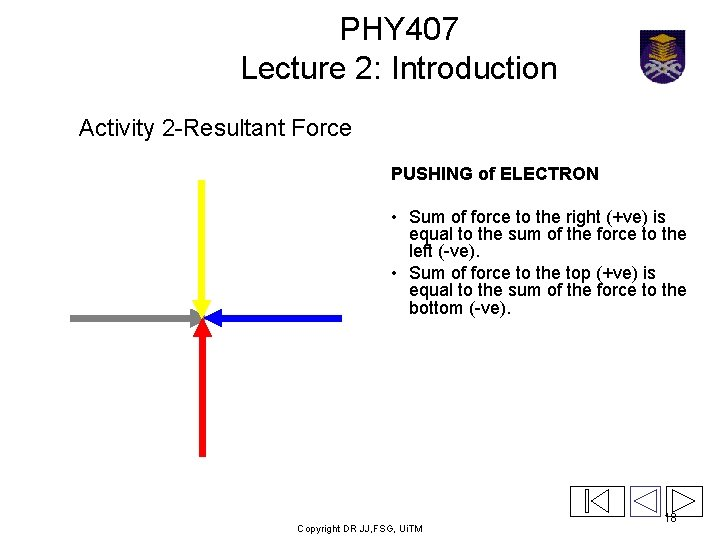PHY 407 Lecture 2: Introduction Activity 2 -Resultant Force PUSHING of ELECTRON • Sum of force to the right (+ve) is equal to the sum of the force to the left (-ve). • Sum of force to the top (+ve) is equal to the sum of the force to the bottom (-ve). Copyright DR JJ, FSG, Ui. TM 18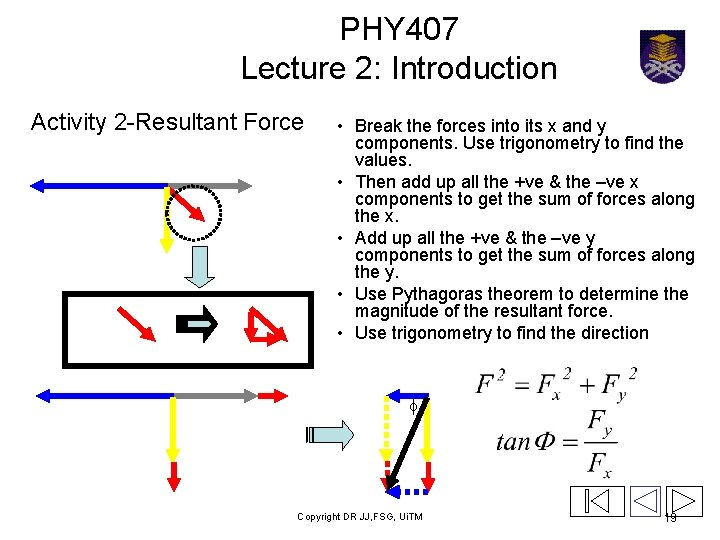PHY 407 Lecture 2: Introduction Activity 2 -Resultant Force • Break the forces into its x and y components. Use trigonometry to find the values. • Then add up all the +ve & the –ve x components to get the sum of forces along the x. • Add up all the +ve & the –ve y components to get the sum of forces along the y. • Use Pythagoras theorem to determine the magnitude of the resultant force. • Use trigonometry to find the direction f Copyright DR JJ, FSG, Ui. TM 19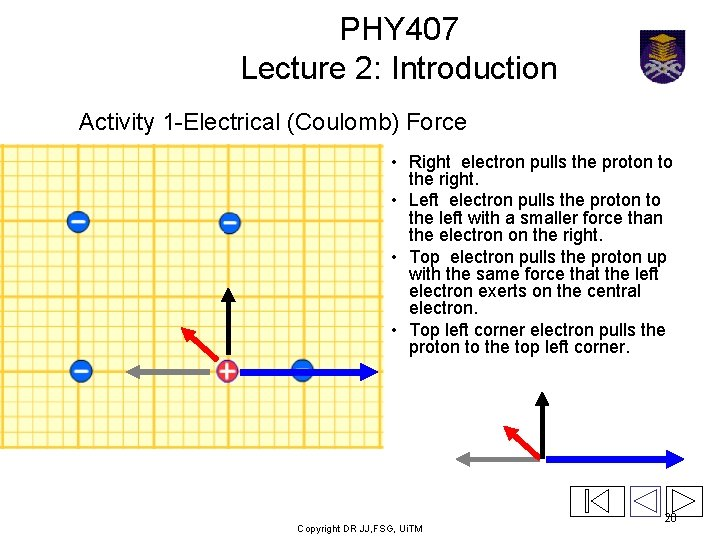PHY 407 Lecture 2: Introduction Activity 1 -Electrical (Coulomb) Force • Right electron pulls the proton to the right. • Left electron pulls the proton to the left with a smaller force than the electron on the right. • Top electron pulls the proton up with the same force that the left electron exerts on the central electron. • Top left corner electron pulls the proton to the top left corner. Copyright DR JJ, FSG, Ui. TM 20